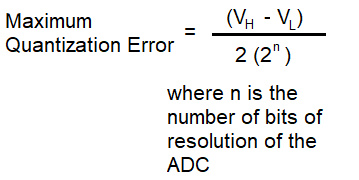﻿ Maximum Quantization Error for ADCs Calculator ﻿# Maximum Quantization Error for ADCs CalculatorHigh Voltage, VH: volts millivolts microvolts Low Voltage, VL: volts millivolts microvolts Number of ADC Bits:

This maximum quantization calculator for ADCs calculates the maximum quantization error for an ADC.

So ADCs do not create exact accurate representation of real-life quantitative values. This is because ADCs are limited by the number of bits, or their resolution. While it is true that the greater the number of bits that compose an ADC causes a more accurate representation of the real-world quantity, the number of bits is still a finite number that can never create an exact replication of the real-world value.

So the maximum quantization error highlights the greatest difference there could be between the true (real-world value) and the reported value by the ADC.

As shown above, the formula to calculate the maximum quantization error is, Maximum quantization error = (VH - VL)/2(2n), where n is the number of bits of resolution of the ADC.

So, as you can see, based on the formula, the greater the voltage range there is between the high voltage, VH, and the low voltage, VL, the greater the possibility for errors.

You can also see that the smaller the number of bits of resolution of the ADC causes a greater quantization error. If the ADC has more bits of resolution, then this translates into a smaller quantization error, which makes sense because more bits causes more resolution, which causes more accuracy.

To use this calculator, a user simply enters in 3 values, VH, VL, and the number of bits of resolution of the ADC, and clicks 'Submit'. The resultant value will then be shown in units volts and either millivolts or microvolts.

VH must be greater than VL, or an error will occur.

The number of bits of resolution of the ADC must be a positive integer, or an error will occur.

Again, the maximun quantization error can help us to know the maximum difference that may exist between the true value and the reported value of an ADC output.

Related Resources

﻿